WEBINAR
Numerical Analysis of Magnetic Pulse Welding Process
Thursday, October 5, 2023
Time
SESSION 1
SESSION 2
CEST (GMT +2)
03:00 PM
08:00 PM
EDT (GMT -4)
09:00 AM
02:00 PM
HOME / Applications / Numerical simulation of graphite crucible at different frequency

# Numerical simulation of graphite crucible at different frequency

Used Tools:## Induction heating

Induction heating is highly effective because it generates heat inside the material almost immediately without physically touching it, it has high-power density and adjustable penetration depths. Compared to other heating techniques, it takes less time to heat and is more efficient and accurate.

Induction heating is a direct application of two laws of physics: the Lenz law and the Joule law. When immersed in a variable magnetic field (generated by an induction coil or inductor), any substance that conducts electricity carries the electrical current induced, also called Eddy currents. Per the Joule effect, the movement of the electrons creating these currents dissipates the heat in the substance where they were generated.

In practical terms, refer to Figure 1,induction heating involves putting a part (usually made of electrical conductive material) inside a magnetic field, which is kept variable with an "inductor coil", which itself is connected to a power source and a capacitor bank, and the assembly forms an oscillating circuit at "resonance" frequency.The quality of energy transmission to the part being treated depends on the placement of the inductors and the parts (connection, respective lengths), the power frequency and the skin effect, which characterizes the distribution of currents induced in the part on the surface or at the core. The higher the frequency, the more the induced currents concentrate on the surface, which determines a "penetration" depth (skin thickness).

This technology started being introduced for industrial applications in the 1960s. Since then, it has constantly been developed and used for innovative solutions.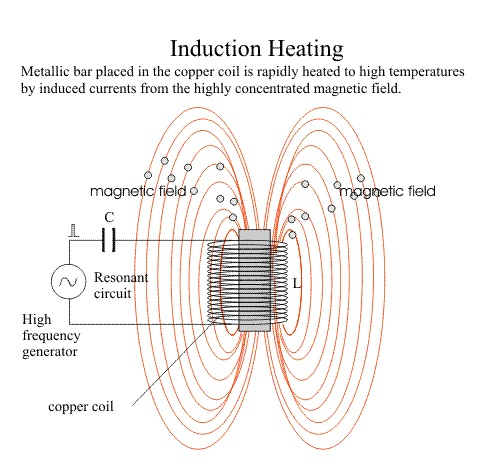Figure 1 - Induction heating principle

## Multiphysics simulation using EMS for Solidworks

Induction heating is multi-physics problemi.e combination of electromagnetism and heattransfer. These physics are nonlinearly coupled with each other due to temperature dependentmaterial properties. Mathematical modeling of electromagnetism and heat transfer is done by well known Maxwell equations and classical heat transfer equation respectively. Field equations are solved by using finite element method in EMS.

EMSensures a Multi-physics simulation environment by the capability of coupling between electro-magnetic- thermal field. In this example, EMS helps to design and optimize the induction coil and graphite crucible for heating application. In EMS, anAC Magnetic simulation coupled to transient thermal analysisis needed.

### Problem description

Induction melting set up comprises the power source, water cooled copper induction coil, graphitecrucible and charge. Power source supplies high frequency current to the induction coil togenerate varying magnetic field and this heats the crucible .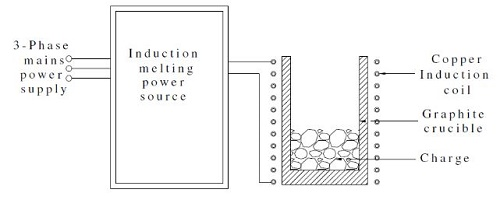Figure 2 - Schematic of induction heating system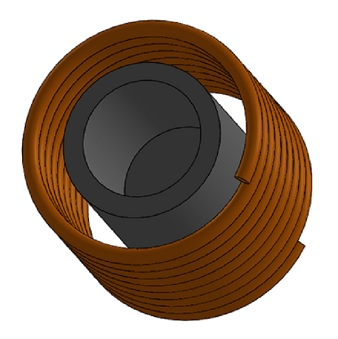Figure 3 - 3D CAD of simulated model

## Simulation Setup

After creating anAC Magnetic studycoupled totransient thermal analysis inEMS, four important steps shall always be followed:

1. apply the proper material for all solid bodies
2. apply the necessary electromagnetic inputs
3. apply the necessary thermal inputs
4. Mesh and Run the simulation

### Materials

Belowin table 1 arethe properties of materials used for simulation. Maxwell equations are solved in complete solution domain (induction coil, graphite crucible, air). Heat transfer analysis is done only in graphite crucible, because induction coil which is water cooled is assumed to be always at room temperature. Electrical conductivity, thermal conductivity and specific heat of graphite are all temperature depend (Figure 4,5,6).

Table1 -  Material properties

 Material Relative Permeability Electrical Conductivity Mass Density (Kg/m^3) Copper 1 5.9980e+7 8900 Graphite 1 Temperature dependent 2500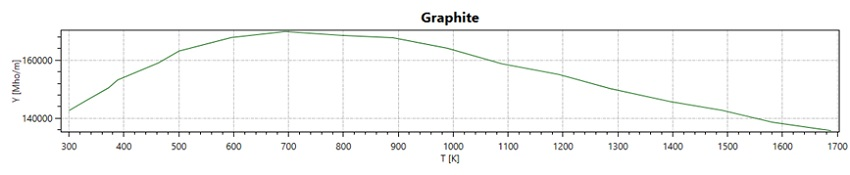Figure 2 - Curve of electrical conductivity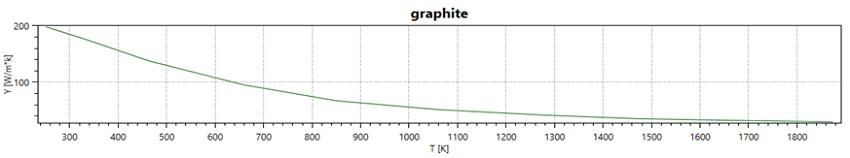Figure 3 - Curve of thermal conductivity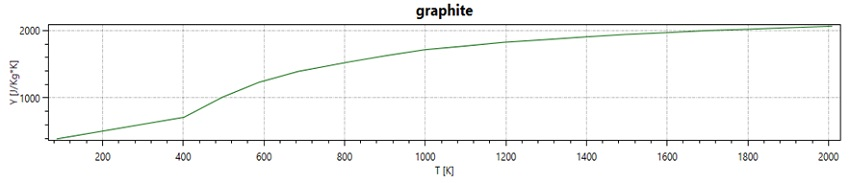Figure 4 - Curve of specific heat

Electromagnetic inputsIn this study, only asolid coil is defined as electromagnetic input as shown in Table 2.

Table 2 - Coil information

 Number of turns RMS Current Solid Coil 9 707.21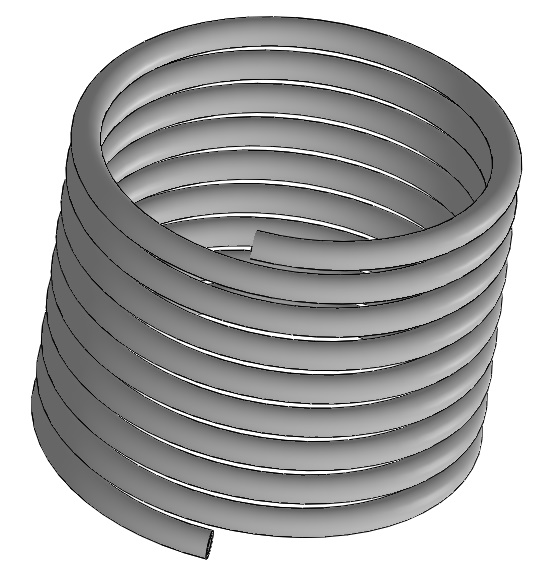Figure 5 - Solid coil used in the simulation

### Thermal inputs and initial conditions

Initial and boundary condition for Heat transfer are listed in table 2.

Table 3 -Thermal parameters

 Boundary conditions Value Initial temperature 303 K Convection coefficient (h) 10 (W/m^2*K) Emissivity (Graphite surface) 0.7

## Meshing

Meshing is a very crucial step in the design analysis. EMS estimates a global element size for the model taking into consideration its volume, surface area, and other geometric details. The size of the generated mesh (number of nodes and elements) depends on the geometry and dimensions of the model, element size, mesh tolerance, and mesh control. In the early stages of design analysis where approximate results may suffice, you can specify a larger element size for a faster solution. For a more accurate solution, a smaller element size may be required.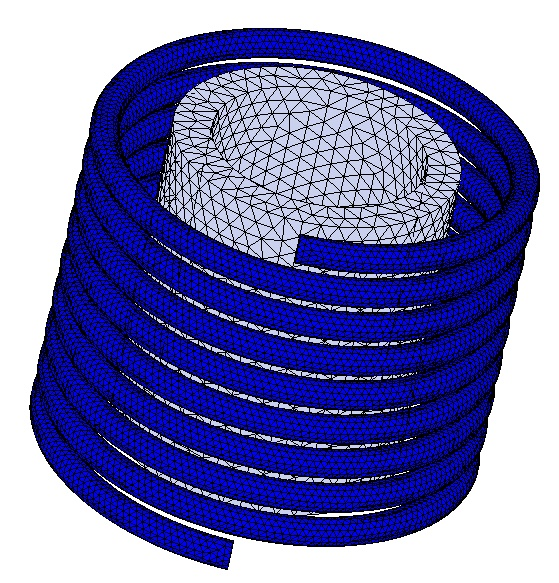Figure 6 - Meshed model

## Results

After running AC magnetic simulation coupled to transient thermal analysis, electromagnetic and thermal results are generated by EMS. Magnetic flux density, field intensity, eddy current, Induced heat power can be plotted with different 3D format. Results table containing the circuit parameters is also generated.
Figures below show the magnetic field intensity respectively at 1kHz, 5kHz, 9kHz. Figure 9 shows the temperature evolution at a point on the surface of the graphite.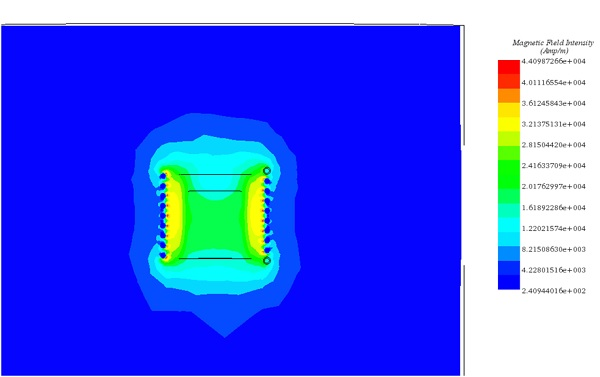Figure 7 - Magnetic field at t=0s for frequency= 1Khz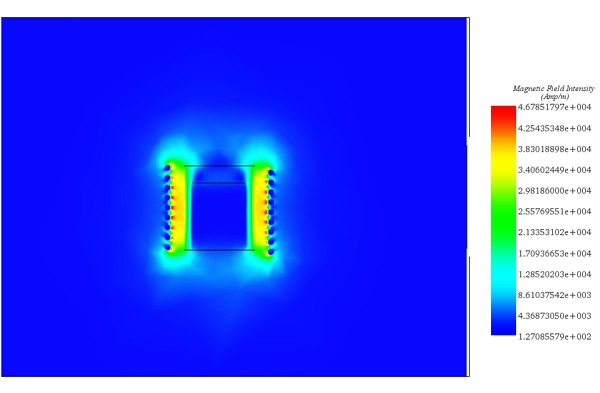Figure 8 - Magnetic field at t=0s for frequency= 5kHz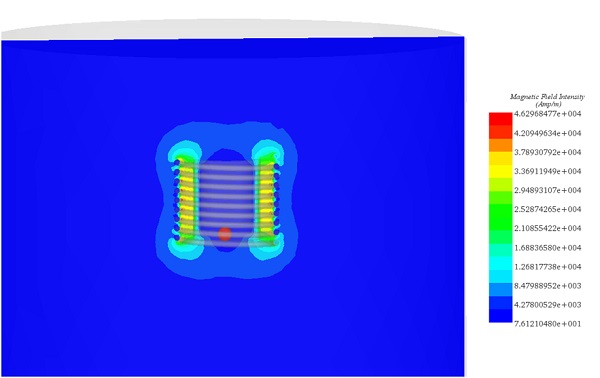Figure 9 - Magnetic field at t=0s for frequency =9kHz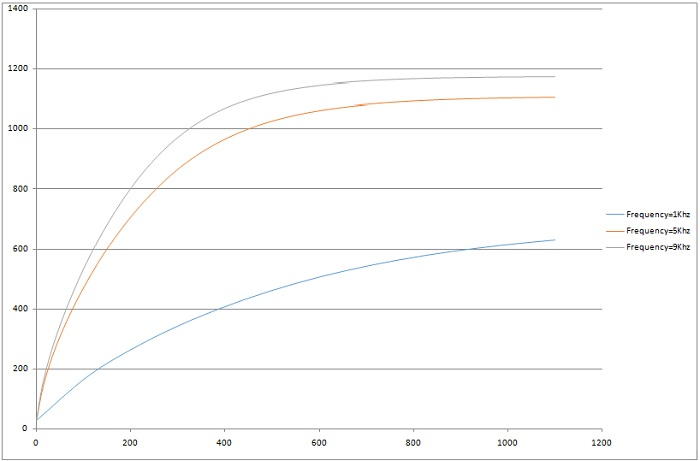Figure 10 - Temperature evolution at specific point

## Conclusion

EMS helps to analyze and compute the induced electromagnetic power in the crucible at different frequencies which allows better understanding of heat transfer in the crucible.  Using this method, crucible wall thickness and induction coil are analyzed which can help to select optimum crucible thickness and an effective cooling system.

## References

B. Patidar,M.M.Hussain,A. Sharma, A.P. Tiwari.August 2016.TRANSIENT NUMERICAL ANALYSIS OF INDUCTION
HEATING OF GRAPHITE CRUCIBLE AT DIFFERENTFREQUENCY. International Journal of Electromagnetics ( IJEL ), Vol 1, No 1,